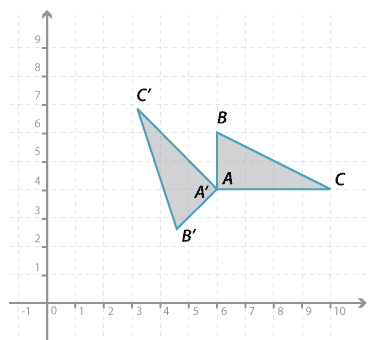#### Question 2$$\triangle ABC$$ can be moved on top of $$\triangle A'B'C'$$ by a rotation in an anticlockwise direction of:

 45 ° 135 ° 90 ° 180 °

$$\triangle ABC$$ is congruent to $$\triangle A'B'C'$$.